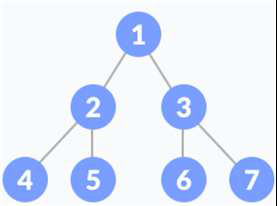# Golang program to traverse a given tree in Inorder Traversal (Recursive).

## Example

Suppose we have the following tree.Inorder Tree Traversal Output − 4 2 5 1 6 3 7

Approach to solve this problem

Step 1 − If the root node of the given tree is nil, then return; else, follow the steps given below.

Step 2 − Traverse the Left sub-tree.

Step 3 − Print the root node data.

Step 4 − Traverse the Right sub-tree.

## Example

Live Demo

package main
import "fmt"
type Node struct {
data int
left *Node
right *Node
}
func (root *Node)InOrderTraversal(){
if root !=nil{
root.left.InOrderTraversal()
fmt.Printf("%d ", root.data)
root.right.InOrderTraversal()
}
return
}
func main(){
tree := Node{1, &Node{2, &Node{4, nil, nil}, &Node{5, nil, nil}}, &Node{3, &Node{6, nil, nil},          &Node{7, nil, nil}}}
fmt.Printf("In Order Traversal of the given tree is: ")
tree.InOrderTraversal()
}

## Output

In Order Traversal of the given tree is: 4 2 5 1 6 3 7